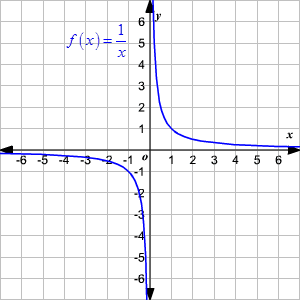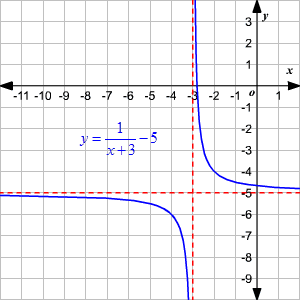# Domain and Range of Rational Functions

The  domain  of a  function $f\left(x\right)$ is the set of all values for which the function is defined, and the  range  of the function is the set of all values that $f$ takes.

A rational function is a function of the form $f\left(x\right)=\frac{p\left(x\right)}{q\left(x\right)}$ , where $p\left(x\right)$ and  $q\left(x\right)$ are polynomials and $q\left(x\right)\ne 0$ .

The domain of a rational function consists of all the real numbers $x$ except those for which the denominator is $0$ . To find these $x$ values to be excluded from the domain of a rational function, equate the denominator to zero and solve for $x$ .

For example, the domain of the parent function $f\left(x\right)=\frac{1}{x}$  is the set of all real numbers except $x=0$ . Or the domain of the function $f\left(x\right)=\frac{1}{x-4}$  is the set of all real numbers except $x=4$ .

Now, consider the function $f\left(x\right)=\frac{\left(x+1\right)\left(x-2\right)}{\left(x-2\right)}$ . On simplification, when $x\ne 2$ it becomes a linear function $f\left(x\right)=x+1$ . But the original function is not defined at $x=2$ . This leaves the graph with a hole when $x=2$ .One way of finding the range of a rational function is by finding the domain of the inverse function.

Another way is to sketch the graph and identify the range.

Let us again consider the parent function $f\left(x\right)=\frac{1}{x}$ . We know that the function is not defined when $x=0$ .

As $x\to 0$  from either side of zero, $f\left(x\right)\to \infty$ . Similarly, as $x\to ±\infty ,f\left(x\right)\to 0$ .The graph approaches $x$ -axis as $x$ tends to positive or negative infinity, but never touches the $x$ -axis. That is, the function can take all the real values except $0$ .

So, the range of the function is the set of real numbers except $0$ .

Example 1:

Find the domain and range of the function $y=\frac{1}{x+3}-5$ .

To find the excluded value in the domain of the function, equate the denominator to zero and solve for $x$ .

$x+3=0⇒x=-3$

So, the domain of the function is set of real numbers except $-3$ .

The range of the function is same as the domain of the inverse function. So, to find the range define the inverse of the function.

Interchange the $x$ and $y$ .

$x=\frac{1}{y+3}-5$

Solving for $y$ you get,

$\begin{array}{l}x+5=\frac{1}{y+3}⇒y+3=\frac{1}{x+5}\\ \text{\hspace{0.17em}}\text{\hspace{0.17em}}\text{\hspace{0.17em}}\text{\hspace{0.17em}}\text{\hspace{0.17em}}\text{\hspace{0.17em}}\text{\hspace{0.17em}}\text{\hspace{0.17em}}\text{\hspace{0.17em}}\text{\hspace{0.17em}}\text{\hspace{0.17em}}\text{\hspace{0.17em}}\text{\hspace{0.17em}}\text{\hspace{0.17em}}\text{\hspace{0.17em}}\text{\hspace{0.17em}}\text{\hspace{0.17em}}\text{\hspace{0.17em}}\text{\hspace{0.17em}}\text{\hspace{0.17em}}\text{\hspace{0.17em}}\text{\hspace{0.17em}}\text{\hspace{0.17em}}\text{\hspace{0.17em}}\text{\hspace{0.17em}}\text{\hspace{0.17em}}⇒y=\frac{1}{x+5}-3\end{array}$

So, the inverse function is ${f}^{-1}\left(x\right)=\frac{1}{x+5}-3$ .

The excluded value in the domain of the inverse function can be determined byequating the denominator to zero and solving for $x$ .

$x+5=0⇒x=-5$

So, the domain of the inverse function is the set of real numbers except $-5$ . That is, the range of given function is the set of real numbers except $-5$ .

Therefore, the domain of the given function is $\left\{x\in ℝ\text{\hspace{0.17em}}\text{\hspace{0.17em}}|\text{\hspace{0.17em}}\text{\hspace{0.17em}}x\ne -3\right\}$  and the range is $\left\{y\in ℝ\text{\hspace{0.17em}}\text{\hspace{0.17em}}|\text{\hspace{0.17em}}\text{\hspace{0.17em}}y\ne -5\right\}$ .Example 2:

Find the domain and range of the function $y=\frac{{x}^{2}-3x-4}{x+1}$ .

Use a graphing calculator to graph the function.

When you factor the numerator and cancel the non-zero common factors, the function gets reduced to a linear function as shown.

$\begin{array}{l}y=\frac{\left(x+1\right)\left(x-4\right)}{\left(x+1\right)}\\ \text{\hspace{0.17em}}\text{\hspace{0.17em}}\text{\hspace{0.17em}}\text{\hspace{0.17em}}=\frac{\overline{)\left(x+1\right)}\left(x-4\right)}{\overline{)\left(x+1\right)}}\\ \text{\hspace{0.17em}}\text{\hspace{0.17em}}\text{\hspace{0.17em}}\text{\hspace{0.17em}}=x-4\end{array}$

So, the graph is a linear one with a hole at $x=-1$ .Use the graph to identify the domain and the range.

The function is not defined for $x=-1$ . So, the domain is $\left\{x\in ℝ\text{\hspace{0.17em}}\text{\hspace{0.17em}}|\text{\hspace{0.17em}}\text{\hspace{0.17em}}x\ne -1\right\}$  or $\left(-\infty ,-1\right)\cup \left(-1,\infty \right)$ .

The range of the function is .

For $x\ne -1$ , the function simplifies to $y=x-4$ . The function is not defined at $x=-1$  or the function does not take the value $-1-4=-5$ . That is, $k=-5$ .

Therefore, the range of the function is $\left\{y\in ℝ\text{\hspace{0.17em}}\text{\hspace{0.17em}}|\text{\hspace{0.17em}}\text{\hspace{0.17em}}y\ne -5\right\}$  or $\left(-\infty ,-5\right)\cup \left(-5,\infty \right)$ .

## Asymptotes of a rational function:

An asymptote is a line that the graph of a function approaches, but never touches. In the parent function $f\left(x\right)=\frac{1}{x}$ , both the  $x$ - and  $y$ -axes are asymptotes. The graph of the parent function will get closer and closer to but never touches the asymptotes.

To find the vertical asymptote of a rational function, equate the denominator to zero and solve for $x$ .

If the degree of the polynomial in the numerator is less than that of the denominator, then the horizontal asymptote is the $x$ -axis or $y=0$ .

The function $f\left(x\right)=\frac{a}{x},a\ne 0$  has the same domain, range and asymptotes as $f\left(x\right)=\frac{1}{x}$ .

Now, the graph of the function $f\left(x\right)=\frac{a}{x-b}+c,a\ne 0$  is a hyperbola, symmetric about the point $\left(b,c\right)$ . The vertical asymptote of the function is $x=b$ and the horizontal asymptote is $y=c$ .

Considering a more general form, the function $f\left(x\right)=\frac{ax+b}{cx+d}$  has the vertical asymptote at $x=-\frac{d}{c}$ and the horizontal asymptote at $y=\frac{a}{c}$ . More generally, if both the numerator and the denominator have the same degree, then horizontal asymptote would be $y=k$ where $k$ is the ratio of the leading coefficient of the numerator to that of the denominator.

If the degree of the denominator is one less than that of the numerator, then the function has a slanting asymptote.

Example 3:

Find the vertical and horizontal asymptotes of the function $f\left(x\right)=\frac{5}{x-1}$ .

To find the vertical asymptote, equate the denominator to zero and solve for $x$ .

$x-1=0⇒x=1$

So, the vertical asymptote is $x=1$

Since the degree of the polynomial in the numerator is less than that of the denominator, the horizontal asymptote is $y=0$ .